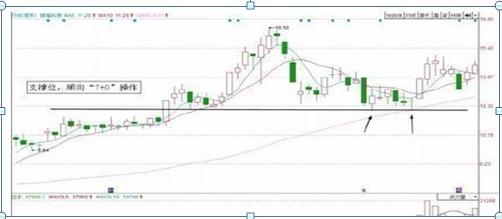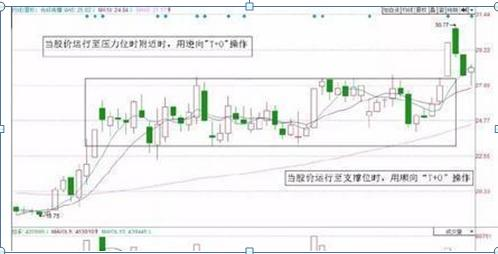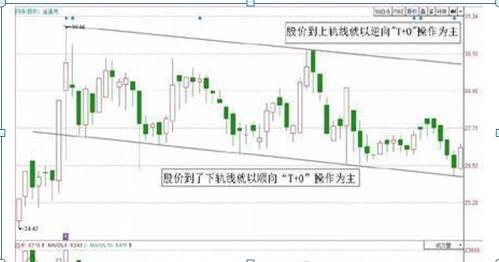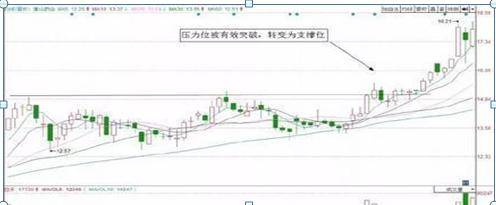# 在股票市场10多年了(长达10年持有一只股票)“没想到,又跌了。”我苦笑说,“大盘当时仅仅出现一波小幅跳水,但中体产业却立刻跌到了18.48元,跌幅接近8%,前两笔资金全部被套。我又看了一眼大盘,认定应该没什么问题,就把最后一笔资金买了进去,在18.52元成交,我心里还想,这是最后一次急跌。”1、果断(洗盘早盘跳水就补仓)

2、不贪心(边拉升，边分批T出来)

3、不要做反(做反的示意图，追涨补回落割)“T+0”的实战技巧：关键点位做T“T+0”交易的技巧在于其主要是以技术面和盘口观察为依据，并且以阻力位、支撑位、时间点、量能变化等作为买卖依据。因此，本节所讲的利用支撑位，压力位做“T+0”，实则是根据股价走势判断买入卖出时机，从而更好利用“T+0”这个操作方法。

V1:=(C*2+H+L)/4*10;V2:=EMA(V1,13)-EMA(V1,34); V3:=EMA(V2,5); V4:=2*(V2-V3)*5.5;

V5:=(HHV(INDEXH,8)-INDEXC)/(HHV(INDEXH,8)-LLV(INDEXL,8))*8;

V6:=EMA(3*V5-2*SMA(V5,18,1),5);

V7:=(INDEXC-LLV(INDEXL,8))/(HHV(INDEXH,8)-LLV(INDEXL,8))*10;

V8:=(INDEXC*2+INDEXH+INDEXL)/4;V9:=EMA(V8,13)-EMA(V8,34);

VA:=EMA(V9,3);VB:=(V9-VA)/2;

V11:=3*SMA((C-LLV(L,55))/(HHV(H,55)-LLV(L,55))*100,5,1)-2*SMA(SMA((C-LLV(L,55))/(HHV(H,55)-LLV(L,55))*100,5,1),3,1);

V12:=(趋势线-REF(趋势线,1))/REF(趋势线,1)*100;

AA:=(趋势线

DRAWTEXT(AA,20,’准备’),COLORCC9900;

BB:= 趋势线<=13 AND V12>13 AND FILTER((趋势线<=13 AND V12>13),10);

DRAWTEXT(BB,5,’买入’),COLORYELLOW;

DRAWTEXT(见顶清仓,90,’逃顶’),COLORYELLOW; CC:=(趋势线>=90 AND V12) AND FILTER((趋势线>=90 AND V12),10);

STICKLINE(大盘资金进场 AND 趋势线<13,0,30,10,0),COLORRED;STICKLINE(大盘资金撤走 AND 趋势线>90,0,30,10,0),COLORGREEN;

STICKLINE(主力进 AND 趋势线<13,0,40,10,0),COLORFF00FF;STICKLINE(主力撤 AND 趋势线>90,0,40,10,0),COLORBLUE;

### 觉得文章有用就打赏一下文章作者

#### 支付宝扫一扫打赏#### 微信扫一扫打赏